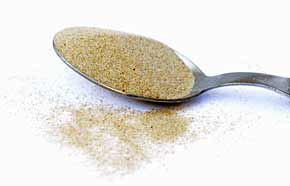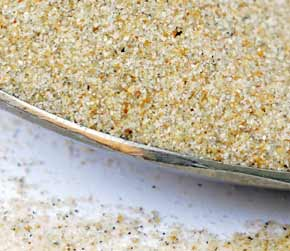﻿ Beach Sand 1 cubic decimeter volume to cubic inches converter

# beach sand conversion

## Amount: 1 cubic decimeter (dm3) of volume Equals: 61.02 cubic inches (cu in - in3) in volume

Converting cubic decimeter to cubic inches value in the beach sand units scale.

TOGGLE :   from cubic inches into cubic decimeters in the other way around.

## beach sand from cubic decimeter to cubic inch Conversion Results:

### Enter a New cubic decimeter Amount of beach sand to Convert From

* Whole numbers, decimals or fractions (ie: 6, 5.33, 17 3/8)
* Precision is how many numbers after decimal point (1 - 9)

Enter Amount :
Decimal Precision :

CONVERT :   between other beach sand measuring units - complete list.

Conversion calculator for webmasters.

## Beach sand weight vs. volume units

Beach sand has quite high density, it's heavy and it easily leaks into even tiny gaps or other opened spaces. No wonder it absorbs and conducts heat energy from the sun so well. However, this sand does not have the heat conductivity as high as glass does, or fireclay and firebricks, or dense concrete. A fine beach sand in dry form was used for taking these measurements.Convert beach sand measuring units between cubic decimeter (dm3) and cubic inches (cu in - in3) but in the other reverse direction from cubic inches into cubic decimeters.

 conversion result for beach sand: From Symbol Equals Result To Symbol 1 cubic decimeter dm3 = 61.02 cubic inches cu in - in3

# Converter type: beach sand measurements

This online beach sand from dm3 into cu in - in3 converter is a handy tool not just for certified or experienced professionals.

First unit: cubic decimeter (dm3) is used for measuring volume.
Second: cubic inch (cu in - in3) is unit of volume.

## beach sand per 61.02 cu in - in3 is equivalent to 1 what?

The cubic inches amount 61.02 cu in - in3 converts into 1 dm3, one cubic decimeter. It is the EQUAL beach sand volume value of 1 cubic decimeter but in the cubic inches volume unit alternative.

How to convert 2 cubic decimeters (dm3) of beach sand into cubic inches (cu in - in3)? Is there a calculation formula?

First divide the two units variables. Then multiply the result by 2 - for example:
61.023744094732 * 2 (or divide it by / 0.5)

QUESTION:
1 dm3 of beach sand = ? cu in - in3

1 dm3 = 61.02 cu in - in3 of beach sand

## Other applications for beach sand units calculator ...

With the above mentioned two-units calculating service it provides, this beach sand converter proved to be useful also as an online tool for:
1. practicing cubic decimeters and cubic inches of beach sand ( dm3 vs. cu in - in3 ) measuring values exchange.
2. beach sand amounts conversion factors - between numerous unit pairs variations.
3. working with mass density - how heavy is a volume of beach sand - values and properties.

International unit symbols for these two beach sand measurements are:

Abbreviation or prefix ( abbr. short brevis ), unit symbol, for cubic decimeter is:
dm3
Abbreviation or prefix ( abbr. ) brevis - short unit symbol for cubic inch is:
cu in - in3

### One cubic decimeter of beach sand converted to cubic inch equals to 61.02 cu in - in3

How many cubic inches of beach sand are in 1 cubic decimeter? The answer is: The change of 1 dm3 ( cubic decimeter ) volume unit of beach sand measure equals = to volume 61.02 cu in - in3 ( cubic inch ) as the equivalent measure within the same beach sand substance type.

In principle with any measuring task, switched on professional people always ensure, and their success depends on, they get the most precise conversion results everywhere and every-time. Not only whenever possible, it's always so. Often having only a good idea ( or more ideas ) might not be perfect nor good enough solution. If there is an exact known measure in dm3 - cubic decimeters for beach sand amount, the rule is that the cubic decimeter number gets converted into cu in - in3 - cubic inches or any other beach sand unit absolutely exactly.

Conversion for how many cubic inches ( cu in - in3 ) of beach sand are contained in a cubic decimeter ( 1 dm3 ). Or, how much in cubic inches of beach sand is in 1 cubic decimeter? To link to this beach sand cubic decimeter to cubic inches online converter simply cut and paste the following.
The link to this tool will appear as: beach sand from cubic decimeter (dm3) to cubic inches (cu in - in3) conversion.

I've done my best to build this site for you- Please send feedback to let me know how you enjoyed visiting.